# Siegel method

A method for investigating the arithmetical properties of the values assumed at algebraic points by \$E\$-functions that satisfy linear differential equations with coefficients in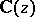; first proposed by C.L. Siegel .

An entire function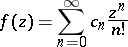is called an-function if all its coefficientsbelong to an algebraic field of finite degree (cf. Algebraic number; Field), if for everythe maximum modulus of the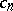isand if there exists a sequence of rational integers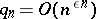such thatis an algebraic integer for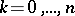. Examples are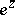,and the Bessel function(cf. Bessel functions).

Let, and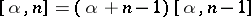,. If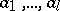andare rational numbers,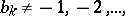and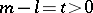, then the function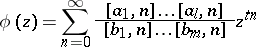is an-function; it satisfies a linear differential equation of orderwith coefficients in.

Siegel's main result pertains to the values of the function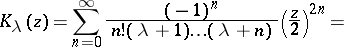whereis the Bessel function. Ifis a rational number,then for any algebraic number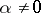the numbers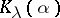andare algebraically independent over(cf. Algebraic independence).

In 1949 Siegel presented his method in a general setting, but the conditions that had to be imposed on the-functions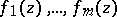so that their values could be assumed algebraically independent proved to be very hard to check. He was therefore unable to achieve any concrete new results.

Further development and generalization of Siegel's method should be credited to A.B. Shidlovskii (see ): Letbe-functions which constitute a solution to the system of differential equations(1)

and letbe an algebraic number distinct from zero and from the singular points of the system (1); then thenumbers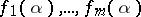are algebraically independent overif and only if the functionsare algebraically independent over. This theorem implies, in particular, that ifare algebraically independent, then all the numbers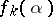are transcendental (cf. Transcendental number); the same holds for all non-zero-points of the functions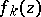distinct from the poles of the system (1), providedis algebraic. The theorem has produced a great number of results concerning specific-functions, and algebraic independence proofs for values of-functions satisfying linear homogeneous and inhomogeneous differential equations of order higher than two. For example, the function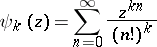satisfies a linear differential equation of orderwith coefficients in; it can be proved that for any algebraic number, thenumbers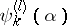,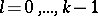;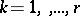, are algebraically independent over.

Under the same conditions, the maximum number of numberswhich are algebraically independent overis equal to the maximum number of functionswhich are algebraically independent over. If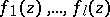are-functions that are algebraically independent overand that satisfy the system (1), then for all points, with the possible exception of finitely many, the numbers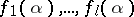are algebraically independent over. In each specific case the exceptional points can actually be determined.

These theorems provide the solution to virtually all problems of a general nature concerning transcendence and algebraic independence of the values of-functions at algebraic points.

Siegel's methods enables one to estimate the measure of algebraic independence of the numbers, thus giving the results a quantitative form. If the functionsare algebraically independent, then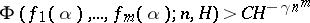, where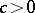is independent ofand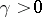depends only onand the degree of the algebraic number.

How to Cite This Entry:
Siegel method. Encyclopedia of Mathematics. URL: http://encyclopediaofmath.org/index.php?title=Siegel_method&oldid=39968
This article was adapted from an original article by Yu.V. Nesterenko (originator), which appeared in Encyclopedia of Mathematics - ISBN 1402006098. See original article